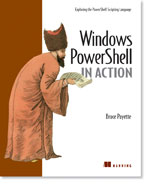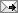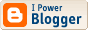# /\/\o\/\/ PowerShelled

This blog has moved to http://ThePowerShellGuy.com Greetings /\/\o\/\/\$AtomFeed = ("Atom.xml")
\$PreviousItems = (" PowerShell Code formatting test for my new Blog "," PowerShell Community Extensions V1.0 "," PowerShell : Access remote eventlogs "," Windows PowerShell Scripting Sweepstakes! "," PowerShell : making Custum Enums "," TechNet Webcast: An Overview of Windows PowerShell "," "Windows PowerShell: TFM" goes "Gold" "," PowerShell : Advanced renaming of files "," PowerShell : Playing with LeapYears "," Download PowerShell 1.0 RTM "," ")

## PowerShell Code formatting test for my new Blog

I'm testing my new blog The PowerShell guy,

I post the same testpost here to compare

Testing codesamples for viewing and copying (do not link here, I will post this item again later after testing , in its final version with a permanent link)

I'm testing with IE7 and Bloglines, if you have problems pasting in the code on the old or new blog please leave a comment,

at the moment it looks like the The PowerShell guy

blog does not have the formatting problems I have here

### convert number to Roman notation

Function as PowerShell code (Pre tag CodeHTMLer)

`# Convert Int to Roman notation# /\/\o\/\/ 2006function format-Roman (\$num  ) {      \$M = [math]::truncate(\$num / 1000)     \$num -= \$M * 1000     \$D = [math]::truncate(\$num / 500)     \$num -=  \$D * 500     \$C = [math]::truncate(\$num / 100)     \$num -=  \$C * 100     \$L = [math]::truncate(\$num / 50)     \$num -=  \$L * 50     \$X = [math]::truncate(\$num / 10)     \$num -=  \$x * 10     \$V = [math]::truncate(\$num / 5)     \$num -=  \$V * 5        \$Roman = "M" * \$M    \$Roman += "D" * \$D    \$Roman += "C" * \$C    \$Roman += "L" * \$L    \$Roman += "X" * \$X    \$Roman += "V" * \$V    \$Roman += "I" * \$num        \$roman = \$roman.replace('DCCCC','CM') # 900    \$roman = \$roman.replace('CCCC','CD') # 400    \$roman = \$roman.replace('LXXXX','XC') # 90    \$roman = \$roman.replace('XXXX','XL') # 40    \$roman = \$roman.replace('VIIII','IX') # 9    \$roman = \$roman.replace('IIII','IV') # 4        Return \$Roman}`

Commandline example (Block Quote)

PoSH>format-Roman 1987
MCMLXXXVII
PoSH>format-Roman 1999
MCMXCIX

PoSH>Update-TypeData C:\PowerShell\TypeData\TypedataInt32.ps1xml
PoSH>(32).toroman()
XXXII

format an Integer in Roman notation

Type extension as XML (convert whitespace, codehtmler)

<?xml version="1.0encoding="utf-8?>
<Types>
<Type>
<Name>System.Int32</Name>
<Members>
<ScriptMethod>
<Name>ToRoman</Name>
<Script>
\$num = \$this

\$M = [math]::truncate(\$num / 1000)
\$num -= \$M * 1000
\$D = [math]::truncate(\$num / 500)
\$num -=  \$D * 500
\$C = [math]::truncate(\$num / 100)
\$num -=  \$C * 100
\$L = [math]::truncate(\$num / 50)
\$num -=  \$L * 50
\$X = [math]::truncate(\$num / 10)
\$num -=  \$x * 10
\$V = [math]::truncate(\$num / 5)
\$num -=  \$V * 5

\$Roman = "M" * \$M
\$Roman += "D" * \$D
\$Roman += "C" * \$C
\$Roman += "L" * \$L
\$Roman += "X" * \$X
\$Roman += "V" * \$V
\$Roman += "I" * \$num

\$roman = \$roman.replace('DCCCC','CM') # 900
\$roman = \$roman.replace('CCCC','CD') # 400
\$roman = \$roman.replace('LXXXX','XC') # 90
\$roman = \$roman.replace('XXXX','XL') # 40
\$roman = \$roman.replace('VIIII','IX') # 9
\$roman = \$roman.replace('IIII','IV') # 4

Return \$roman
</Script>
</ScriptMethod>

</Members>
</Type>
</Types>

* Edit * Added script again formatted by PowerShell analyzer

`# Function Format-Roman# /\/\o\/\/ 006# http://ThePowerShellGuy.com# HTML Formatting Generated with PowerShell Analyzerfunction format-Roman (\$num ) {      \$M = [math]::truncate(\$num / 1000)     \$num -= \$M * 1000     \$D = [math]::truncate(\$num / 500)     \$num -=  \$D * 500     \$C = [math]::truncate(\$num / 100)     \$num -=  \$C * 100     \$L = [math]::truncate(\$num / 50)     \$num -=  \$L * 50     \$X = [math]::truncate(\$num / 10)     \$num -=  \$x * 10     \$V = [math]::truncate(\$num / 5)     \$num -=  \$V * 5        \$Roman = "M" * \$M    \$Roman += "D" * \$D    \$Roman += "C" * \$C    \$Roman += "L" * \$L    \$Roman += "X" * \$X    \$Roman += "V" * \$V    \$Roman += "I" * \$num        \$roman = \$roman.replace('DCCCC','CM') # 900    \$roman = \$roman.replace('CCCC','CD') # 400    \$roman = \$roman.replace('LXXXX','XC') # 90    \$roman = \$roman.replace('XXXX','XL') # 40    \$roman = \$roman.replace('VIIII','IX') # 9    \$roman = \$roman.replace('IIII','IV') # 4        Return \$roman}`

Enjoy, Greetings /\/\o\/\/jv
You have the same problem I have. Can't get the extra space between the lines to go away./\/\o\/\/
Hi JV,

thanks for the reaction,

I had a lot of problems with formatting code also.
on my new blog (CS2.1), the exactly same formatting (all 3 examples), also posted from Live writer seems to work like a charm :

http://thepowershellguy.com/blogs/posh/archive/2006/12/22/code-formatting-test.aspx

also my sidebar does not get messed up, it it great to be able to use the PowerShell analyzer HTML export.

Greetings /\/\o\/\/

<< Home

## Archives

October 2005   November 2005   December 2005   January 2006   February 2006   March 2006   April 2006   May 2006   June 2006   July 2006   August 2006   September 2006   October 2006   November 2006   December 2006

\$Links = ("PowerShell RC1 Docs"," PowerShell RC1 X86"," PowerShell RC1 X64"," Monad GettingStarted guide"," Monad Progamming Guide"," Monad SDK"," Monad videos on Channel 9"," MSH Community Workspace"," scripts.readify.net "," MonadSource"," www.reskit.net"," PowerShell Blog"," Under The Stairs"," computerperformance powershell Home"," proudlyserving"," MSH on wikipedia"," MSHWiki Channel 9"," Keith Hill's Blog"," Precision Computing"," PowerShell for fun"," MSH Memo (Japanese)"," monadblog")